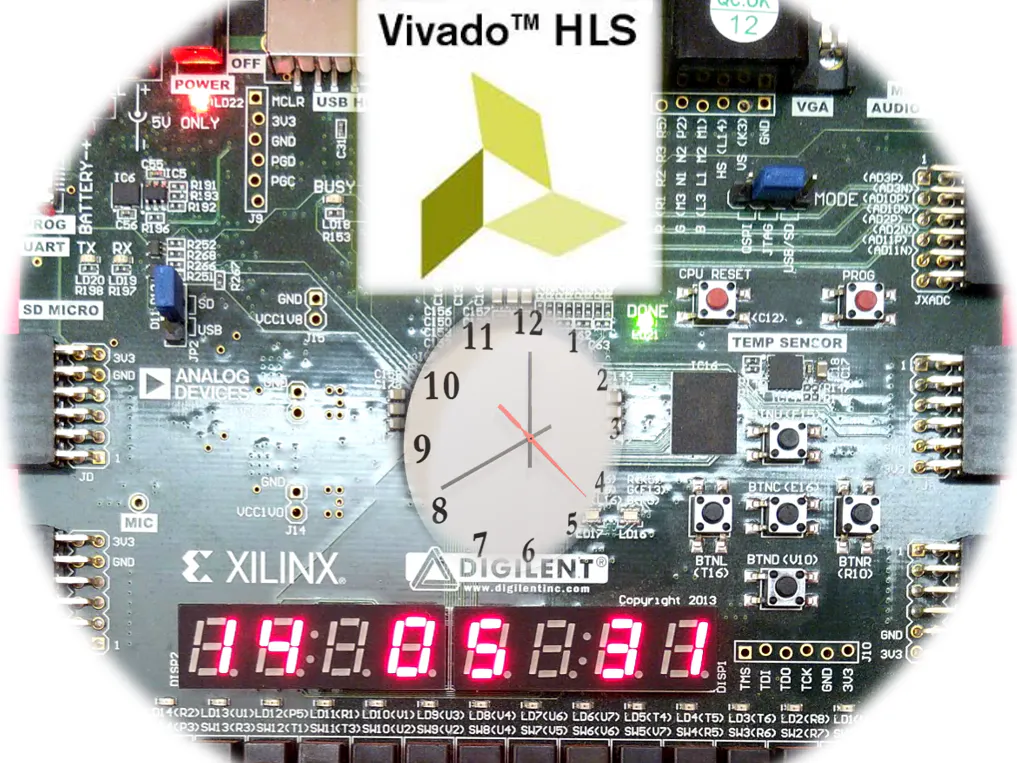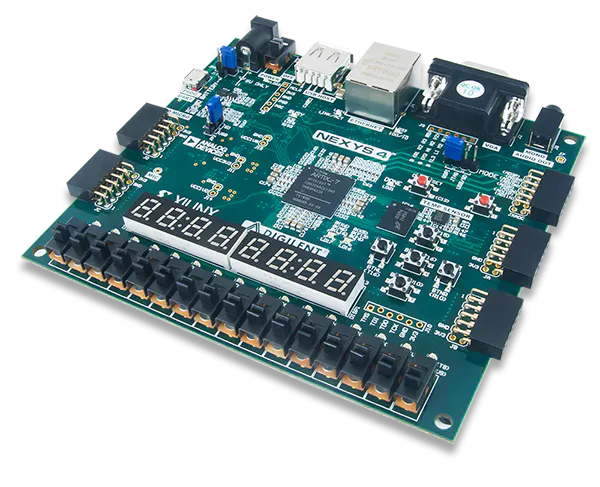# Digital Clock in HLS

The goal of this project is to demonstrate the capability of HLS in designing a digital clock system.

BeginnerFull instructions provided1 hour703## Things used in this project

### Hardware componentsDigilent Nexys 4
×1USB-A to Mini-USB Cable
×1

### Software apps and online servicesXilinx Vivado Design Suite HLx Editions

## Code

### Code snippet #1

Plain text
```bool delay(long long int n) {
#pragma HLS INLINE off
static bool dummy = 0;
for (long long int j = 0; j < n; j++) {
#pragma HLS pipeline
dummy = !dummy;
}
return dummy;
}

void one_second_clock_generator(bool &second) {
#pragma HLS INTERFACE ap_none port=second
#pragma HLS INTERFACE ap_ctrl_none port=return

static bool s = 0;
s=!s;
second = s;
delay(50000000L);
}
```

### Code snippet #2

Plain text
```void debounce(bool pulse, bool &out) {
#pragma HLS INLINE off
static bool out0 = 0;
static bool out1 = 0;
static bool out2 = 0;
static bool state = 0;
if (state == 0) {
out2 = out1;
out1 = out0;
out0 = pulse;
state = 1;
} else {
delay(2500000);
state = 0;
}
out = out0 & out1 & out2;
}
void set_time(
ap_uint<6>  &seconds,
ap_uint<6>  &minutes,
ap_uint<5>  &hours,
bool        set_second,
bool        set_minute,
bool        set_hour)
{
//--------------------------------------------------
static bool second_state = 0;
if (second_state == 0 && set_second == 1 ) {
seconds++;
if (seconds == 60) {
seconds = 0;
}
second_state = 1;
}
if (second_state == 1 && set_second == 0 ) {
second_state = 0;
}
//---------------------------------------------------
static bool minute_state = 0;
if (minute_state == 0 && set_minute == 1 ) {
minutes++;
if (minutes == 60) {
minutes = 0;
}
minute_state = 1;
}
if (minute_state == 1 && set_minute == 0 ) {
minute_state = 0;
}
//----------------------------------------------------
static bool hour_state = 0;
if (hour_state == 0 && set_hour == 1) {
hours++;
if (hours == 24) {
hours = 0;
}
hour_state = 1;
}
if (hour_state == 1 && set_hour == 0) {
hour_state = 0;
}
//----------------------------------------------------
}

void clock_ticking(
ap_uint<5> &hours,
ap_uint<6> &minutes,
ap_uint<6> &seconds)
{

seconds++;
if (seconds == 60) {
seconds = 0;
minutes++;
if (minutes == 60) {
minutes = 0;
hours++;
if (hours == 24)
hours = 0;
}
}
}

void digital_clock(
bool        set_time_sw,
bool       &set_time_led,

bool        set_second,
bool        set_minute,
bool        set_hour,

bool        one_second_delay,

ap_uint<6>  &seconds_out,
ap_uint<6>  &minutes_out,
ap_uint<5>  &hours_out

)
{
#pragma HLS INTERFACE ap_none port=set_time_sw
#pragma HLS INTERFACE ap_none port=set_time_led
#pragma HLS INTERFACE ap_none port=set_minute
#pragma HLS INTERFACE ap_none port=set_hour

#pragma HLS INTERFACE ap_none port=seconds_out
#pragma HLS INTERFACE ap_none port=minutes_out
#pragma HLS INTERFACE ap_none port=hours_out

#pragma HLS INTERFACE ap_ctrl_none port=return

static ap_uint<6> seconds = 0;
static ap_uint<6> minutes = 0;
static ap_uint<5> hours   = 0;

ap_uint<8> segment_data;
ap_uint<8> segment_enable;

static bool state_clock = 0;

bool        one_second = one_second_delay;
bool        set_time_flag = set_time_sw;

if (one_second==1&&set_time_flag==0&&state_clock==0) {
clock_ticking(hours, minutes, seconds);
state_clock = 1;
}

if (one_second==0&&set_time_flag==0&&state_clock==1) {
state_clock = 0;
}

if (set_time_flag == 1) {
bool set_minute_debounce;
bool set_hour_debounce;
bool set_second_debounce;

debounce (set_minute, set_minute_debounce);
debounce (set_hour, set_hour_debounce);
debounce (set_second, set_second_debounce);
set_time(seconds, minutes, hours, set_second_debounce, set_minute_debounce, set_hour_debounce);

}

seconds_out = seconds;
minutes_out = minutes;
hours_out   = hours;

set_time_led = set_time_sw;

}
```

### Code snippet #3

Plain text
```#include <ap_int.h>

const ap_uint<8> seven_segment_code = {
0b11000000,
0b11111001,
0b10100100,
0b10110000,
0b10011001,
0b10010010,
0b10000010,
0b11111000,
0b10000000,
0b10010000
};

bool delay(long long int n) {
#pragma HLS INLINE off
static bool dummy = 0;
for (long long int j = 0; j < n; j++) {
#pragma HLS pipeline
dummy = !dummy;
}
return dummy;
}

void seven_segment_display(
ap_uint<5> hours,
ap_uint<6> minutes,
ap_uint<6> seconds,
ap_uint<8> &seven_segment_data,
ap_uint<8> &seven_segment_enable)
{
#pragma HLS INTERFACE ap_none port=hours
#pragma HLS INTERFACE ap_none port=minutes
#pragma HLS INTERFACE ap_none port=seconds
#pragma HLS INTERFACE ap_none port=seven_segment_data
#pragma HLS INTERFACE ap_none port=seven_segment_enable
#pragma HLS INTERFACE ap_ctrl_none port=return

ap_uint<4> second_digit_1 = seconds%10;
ap_uint<4> second_digit_2 = seconds/10;

ap_uint<4> minute_digit_1 = minutes%10;
ap_uint<4> minute_digit_2 = minutes/10;

ap_uint<4> hours_digit_1 = hours%10;
ap_uint<4> hours_digit_2 = hours/10;

ap_uint<8> segment_data;
ap_uint<8> segment_enable;

static ap_uint<3> state = 0;
switch (state) {
// second
case 0:
segment_data   = seven_segment_code[second_digit_1];
segment_enable = 0b11111110;
delay(250000L);
state = 1;
break;
case 1:
segment_data   = seven_segment_code[second_digit_2];
segment_enable = 0b11111101;
state = 2;
delay(250000L);
break;
// minutes
case 2:
segment_data   = seven_segment_code[minute_digit_1];
segment_enable = 0b11110111;
state = 3;
delay(250000L);
break;
case 3:
segment_data   = seven_segment_code[minute_digit_2];
segment_enable = 0b11101111;
state = 4;
delay(250000L);
break;
// hours
case 4:
segment_data   = seven_segment_code[hours_digit_1];
segment_enable = 0b10111111;
state = 5;
delay(250000L);
break;
case 5:
segment_data   = seven_segment_code[hours_digit_2];
segment_enable = 0b01111111;
state = 0;
delay(250000L);
break;
default:
segment_data   = seven_segment_code;
segment_enable = 0b11111111;
state = 0;
delay(250000L);
break;
}

seven_segment_data = segment_data;
seven_segment_enable = segment_enable;
}
```